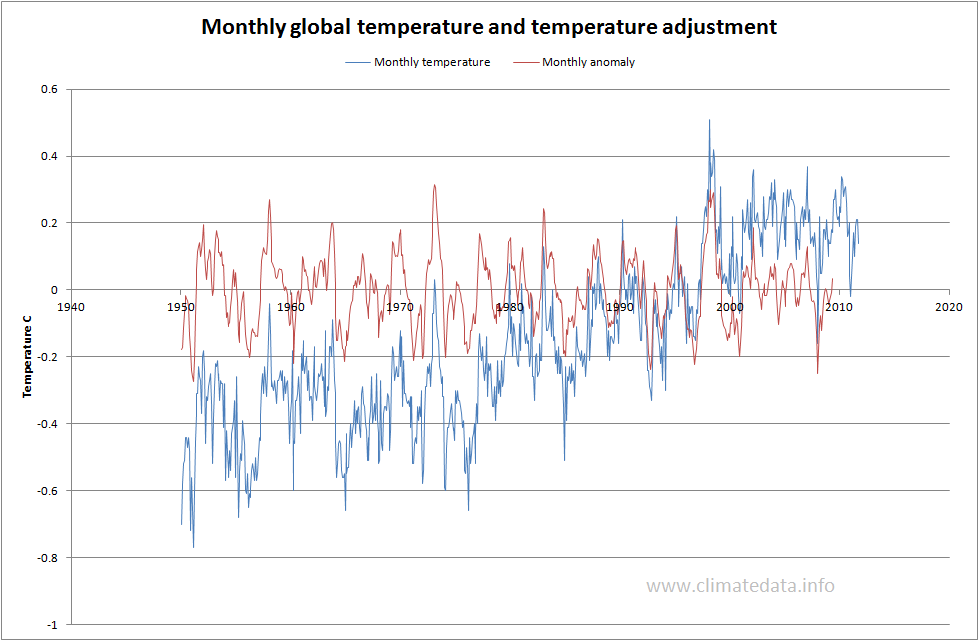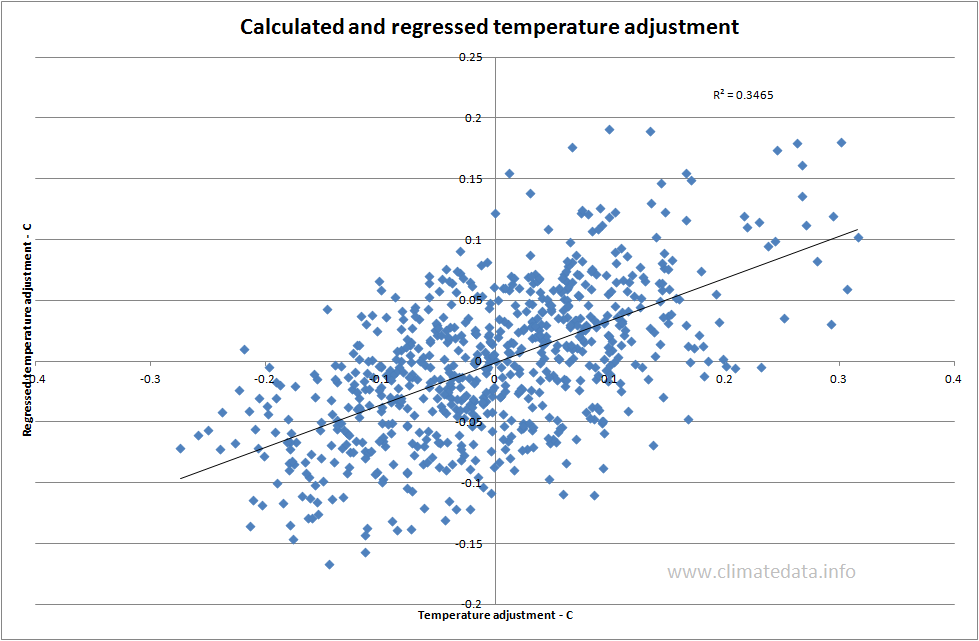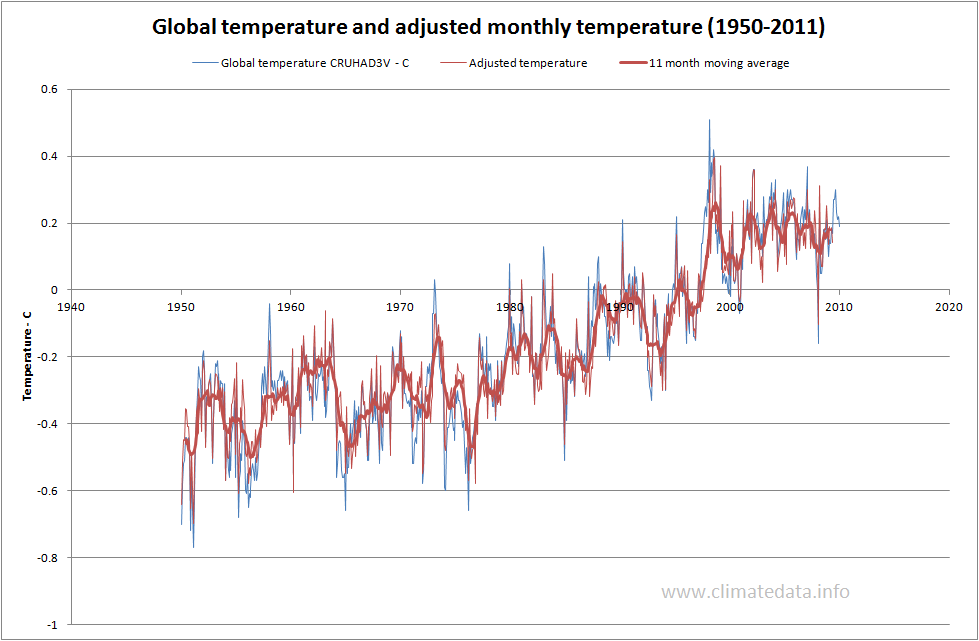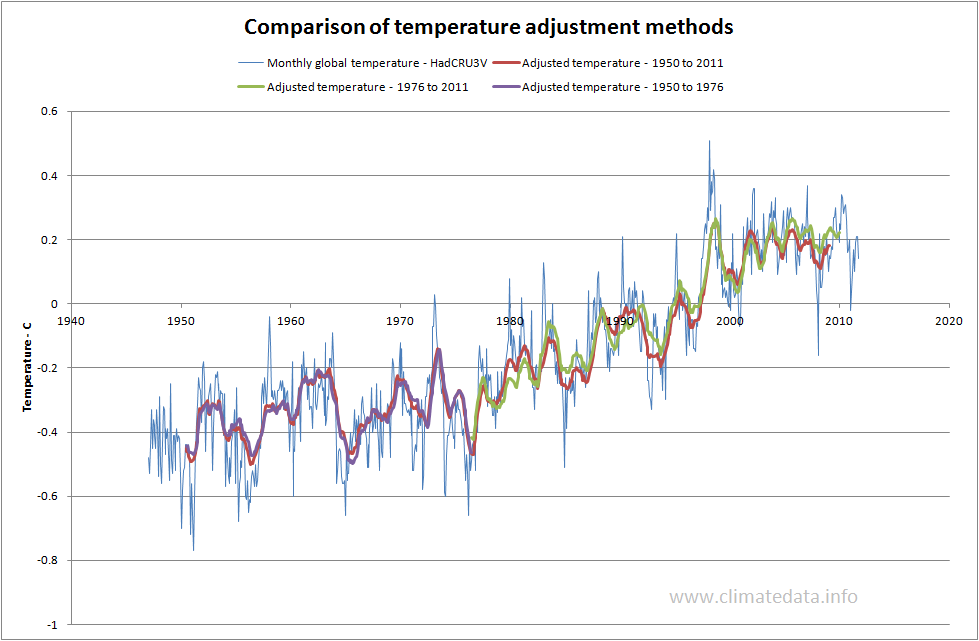# SHORT TERM VARIATIONS IN TEMPERATURE

In a recent paper Foster and Rahmstorf (F and R, Global temperature evolution 1979–2010) examine the influence of three factors which introduce variability to the temperature record: ElNino/Nina, Volcanoes and Total Solar Irradiance(TSI). They chose to represent the El Nino/Nina effect by the Multivariate El Nino Index (MEI), volcanoes by Aerosol Optical Thickness Data and TSI by sunspot number. They describe their regression as "the multiple regression includes a linear time trend, MEI, AOD, TSI and a second-order Fourier series with period 1 yr." Effectively they assumed the temperature perturbation as the difference from a linear trend plus and allowance for seasonal effects. They chose the period 1979 to 2010 as this included two satellite temperature records in addition to three records based on measurements. They concluded that adjusting the temperature records showed that the underlying temperature trend was upwards for the whole period.

However data for all three variables are available from 1950 and, for some variables, much earlier. We therefore examined the effect of the three factors on temperature from 1950 to 2011.

As we could not assume a linear temperature trend for the whole of the period 1950 to 2011 we calculated the adjustment (or perturbation) as the difference between the three month mean and the 60 month mean. The three month mean represented the short term effect and the 60 month mean the underlying temperature trend. We worked only with HadCRU3V global temperature series. The need to use the 60 month mean means that our series is truncated relative to the F and R series.

The first chart shows the adjustment calculated by this method.As can be seen the adjustment clearly represents short term effects such as El Nino and volcanoes.

We then regressed the adjustment against the same three variables as F and R. This chart shows a line trend line between the adjustments and the values calculated from the regression equation.The r2 value was 0.3564, a bit lower than the best of the values found by F and R but covering a longer period.The final plot shows the observed and adjusted temperature series.For the common period between this plot and that of F and R the results are similar, in particular the effect of 1998 El Nino is reduced but on the other hand the trend is not as uniformly positive as theirs; this is probably due to the use of a different measure of temperature adjustment and the fact that there was no assumption of a linear trend. The plot also shows that in the period up to 1976 the temperature was much flatter than from that year on.

To understand better the differences between F and R and our approach we also tried using the difference from an assumed trend to represent the perturbation. For the period 1976 to 2011 we used a linear temperature increase. For the period 1950 to 1976 we assumed a constant temperature (i.e. completely flat trend).For the period 1950 to 1976 the two results are virually identical. For the period 1976 to 2011 the results are similar with the use of a linear trend to define the perturbation giving slightly higher values from 2005 onward. The conclusion is that the results show some sensitivity to the method used to calculate the temperature perturbation. It could be argued that a method such as the one we used, which makes no assumptions relative to the underlying trend, has advantages relative to one which does make such an assumption.

(Note: This was first posted on 17 January and revised and extended on 18 January 2012)
Comments
See Older Posts...